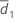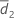# Methods and formulas for common concepts used in Equivalence Test for a 2x2 Crossover Design

## Common notation

All of the calculations for the equivalence test for 2x2 crossover design assume that the treatment order for sequence 1 is the reference treatment followed by the test treatment, and that the order for sequence 2 is the test treatment followed by the reference treatment.

Let Yijk be the response for participant k during period j in sequence i, where i = 1, 2; j = 1, 2; k = 1 ... ni .

If the response for either period is missing for a participant, then the data for that participant is omitted from the calculations.

Let d1k and d2k be defined as follows:

Letand S1 represent the sample mean and the sample standard deviation of d1k, k = 1, ..., n1, given by the following: Letand S2 represent the sample mean and the sample standard deviation of d2k, k = 1, ..., n2 , given by the following:

### Notation

TermDescriptionSample mean of d1k, k = 1, ..., n1
S1Sample standard deviation of d1k, k = 1, ..., n1Sample mean of d2k, k = 1, ..., n2
S2Sample standard deviation of d2k, k = 1, ..., n2

## Degrees of freedom (DF)

### Notation

TermDescription
νDegrees of freedom
n1Number of participants in sequence 1
n2Number of participants in sequence 2

## Equivalence limits

Let k1 be the lower limit that you specify andk2 be the upper limit that you specify. By default, the lower equivalence limit, δ1, is given by:and the upper equivalence limit, δ2, is given by:However, if you select the option to multiply your limits by the reference mean,, then the limits are given by:is the average of the average responses for the two reference periods.

By using this site you agree to the use of cookies for analytics and personalized content.  Read our policy